Courses

Test: MCQs (One or More Correct Option): Momentum and Impulse | JEE Advanced

10 Questions MCQ Test Physics 35 Years JEE Main & Advanced Past year Papers | Test: MCQs (One or More Correct Option): Momentum and Impulse | JEE Advanced

Description
Attempt Test: MCQs (One or More Correct Option): Momentum and Impulse | JEE Advanced | 10 questions in 20 minutes | Mock test for JEE preparation | Free important questions MCQ to study Physics 35 Years JEE Main & Advanced Past year Papers for JEE Exam | Download free PDF with solutions
QUESTION: 1

Two particles of masses m1 and m2 in projectile motion have velocities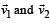respectively at time t = 0. They collide at time to. Their velocities become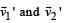at time 2to while still moving in air. The value of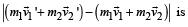Solution:

If we consider the two particles as a system then the external force acting on the system is the gravitational pull (m1 + m2) g.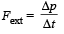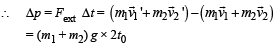QUESTION: 2

Two blocks of masses 10 kg and 4 kg are connected by a spring of negligible mass and placed on a frictionless horizontal surface. An impulse gives a velocity of 14 m/s to the heavier block in the direction of the lighter block. The velocity of the centre of mass is

Solution:

Just after collision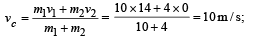Note : Spring force is an internal force, it cannot change the linear momentum of the (two mass + spring) system.
Therefore vc remains the same.

QUESTION: 3

A ball of mass 0.2 kg rests on a vertical post of height 5 m. A bullet of mass 0.01 kg, traveling with a velocity V m/s in a horizontal direction, hits the centre of the ball. After the collision, the ball and bullet travel independently. The ball hits the ground at a distance of 20 m and the bullet at a distance of 100 m from the foot of the post. The velocity V of the bullet is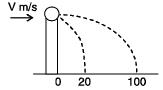Solution:

For vertical motion of bullet or ball
u = 0,  s = 5m,  t = ? ,  a = 10m/s2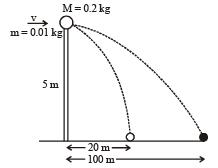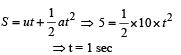For horizontal motion of ball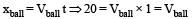For horizontal motion of bullet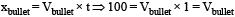Applying conservation of linear momentum during collision, w e get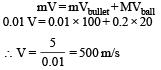QUESTION: 4

A particle of mass m is projected from the ground with an initial speed u0 at an angle α with the horizontal. At the highest point of its trajectory, it makes a completely inelastic collision with another identical particle, which was thrown vertically upward from the ground with the same initial speed u0. The angle that the composite system makes with the horizontal immediately after the collision is

Solution:

Activity B to M for particle thrown upwards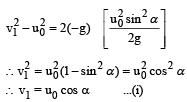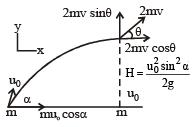Applying conservation of linear momentum in Y-direction
2mv sinθ = mv1 = mu0cosα ...(ii)    [from (i)]
Applying conservation of linear momentum in X-direction
2mv cosθ = mu0 cosα ...(iii)
on dividing (ii) and (iii) we get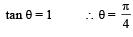*Multiple options can be correct
QUESTION: 5

A ball hits the floor and rebounds after an inelastic collision. In this case

Solution:

(a) is wrong because the momentum of ball  changes in magnitude as well as direction.
(b) is wrong because on collision, some mechanical energy is converted into heat, sound energy.

(c) is correct because for earth + ball system the impact force is an internal force.
(d) is correct.

*Multiple options can be correct
QUESTION: 6

A shell is fired from a cannon with a velocity v (m/sec.) at an angle θ with the horizontal direction. At the highest point in its path it explodes into two pieces of equal mass. One of the pieces retraces its path to the cannon and the speed (in m/sec.) of the other piece immediately after the explosion is

Solution:

As one piece retraces its path, the speed of this piece just after explosion should be v cos θ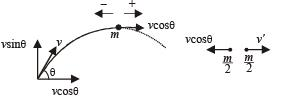(At highest point just after explosion) NOTE THIS STEP
Applying conservation of linear momentum at the highest point;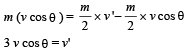*Multiple options can be correct
QUESTION: 7

Two blocks A and B, each of mass m, are connected by a massless spring of natural length L and spring constant K.
The blocks are initially resting on a smooth horizontal floor with the spring at its natural length, as shown in fig.. A third identical block C, also of mass m, moves on the floor with a speed v along the line joining A and B, and collides elastically with A. Then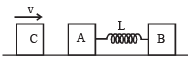Solution:

In situation 1, mass C is moving towards right with velocity v. A and B are at rest.
In situation 2, which is just after the collision of C with A, C stop and A acquires a velocity v. [head-on elastic collision between identical masses]
When A starts moving towards right, the spring suffer a compression due to which B also starts moving towards right. The compression of the spring continues till there is relative velocity between A  and B. When this relative velocity becomes zero, both A and B move with the same velocity v' and the spring is in a state of maximum compression.
Applying momentum conservation in situation 1 and 3,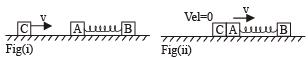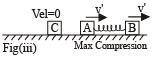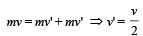∴ K.E. of the system in situation 3 is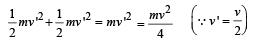This is the kinetic energy possessed by A – B system (since, C is at rest).
Let x be the maximum compression of the spring.
Applying energy conservation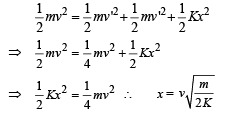*Multiple options can be correct
QUESTION: 8

The balls, having linear momenta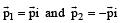undergo a collision in free space. There is no external force acting on the balls. Let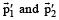be their final momenta. The following option (s) is (are) NOT ALLOWED for any non-zero value of p, a1, a2, b1, b2, c1 and c2.

Solution:

KEY CONCEPT Use law of conservation of linear momentum.
The initial linear momentum of the system is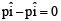Therefore the final linear momentum should also be zero.

Option a :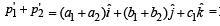Final momentum.
It is given that a1, b1, c1, a2, b2 and c2 have non-zero values. If a1 = x and a2 = – x. Also if b1 = y and b2 = – y then the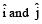components become zero. But the third term having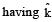component is non-zero. This gives a definite final momentum to the system which violates conservation of linear momentum, so this is an incorrect option.

Option d: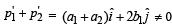because b1 ≠ 0
Following the same reasoning as above this option is also ruled ou

*Multiple options can be correct
QUESTION: 9

A point mass of 1 kg collides elastically with a stationary point mass of 5 kg. After their collision, the 1 kg mass reverses its direction and moves with a speed of 2 ms–1. Which of the following statement(s) is (are) correct for the system of these two masses?

Solution:

According to law of conservation of linear momentum 1 × u1 + 5 × 0 = 1 (–2) + 5 (v2)
⇒ u1 = – 2 + 5 v2 ...(i)

The coefficient of restituition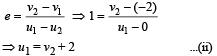On solving (i) & (ii) we get desired results.

*Multiple options can be correct
QUESTION: 10

A particle of mass m is attached to one end of a mass-less spring of force constant k, lying on a frictionless horizontal plane. The other end of the spring is fixed. The particle starts moving horizontally from its equilibrium position at time t = 0 with an initial velocity u0. When the speed of the particle is 0.5 u0, it collides elastically with a rigid wall. After this collision

Solution:

The particle collides elastically with rigid wall. Here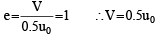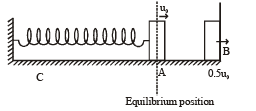i.e. the particle rebounds with the same speed. Therefore the particle will return to its equilibrium position with speed u0. option (a) is correct.
The velocity of the particle becomes 0.5u0 after time t.
Then using the equation V = Vmax cos wt we get 0.5u0 = u0 cos wt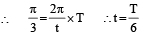The time period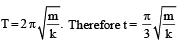The time taken by the particle to pass through the equilibrium for the first time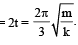Therefore option (b) is incorrect
The time taken for the maximum compression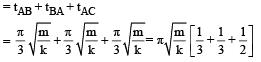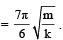Therefore option c is incorrect.
The time taken for particle to pass through the equilibrium position second time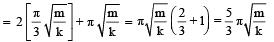option (d) is correct.Use Code STAYHOME200 and get INR 200 additional OFF Use Coupon Code

Track your progress, build streaks, highlight & save important lessons and more!

Similar ContentRelated tests